FreeRDP
Layout.c File Reference
`#include <stdio.h>`
`#include <string.h>`
`#include <stdarg.h>`
`#include <winpr/crt.h>`
`#include <winpr/print.h>`
`#include <winpr/sysinfo.h>`
`#include <winpr/environment.h>`
`#include "wlog.h"`
`#include "wlog/Layout.h"`

Functions

static void WLog_PrintMessagePrefixVA (wLog *log, wLogMessage *message, const char *format, va_list args)

static void WLog_PrintMessagePrefix (wLog *log, wLogMessage *message, const char *format,...)

BOOL WLog_Layout_GetMessagePrefix (wLog *log, wLogLayout *layout, wLogMessage *message)

wLogLayout * WLog_GetLogLayout (wLog *log)

BOOL WLog_Layout_SetPrefixFormat (wLog *log, wLogLayout *layout, const char *format)

wLogLayout * WLog_Layout_New (wLog *log)

void WLog_Layout_Free (wLog *log, wLogLayout *layout)

Variables

const char * WLOG_LEVELS 

Function Documentation

 wLogLayout* WLog_GetLogLayout ( wLog * log )

Here is the call graph for this function: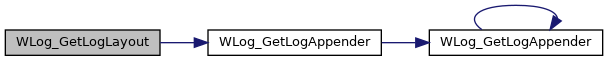Here is the caller graph for this function: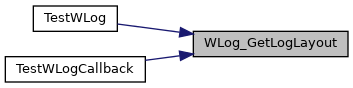void WLog_Layout_Free ( wLog * log, wLogLayout * layout )

Here is the caller graph for this function: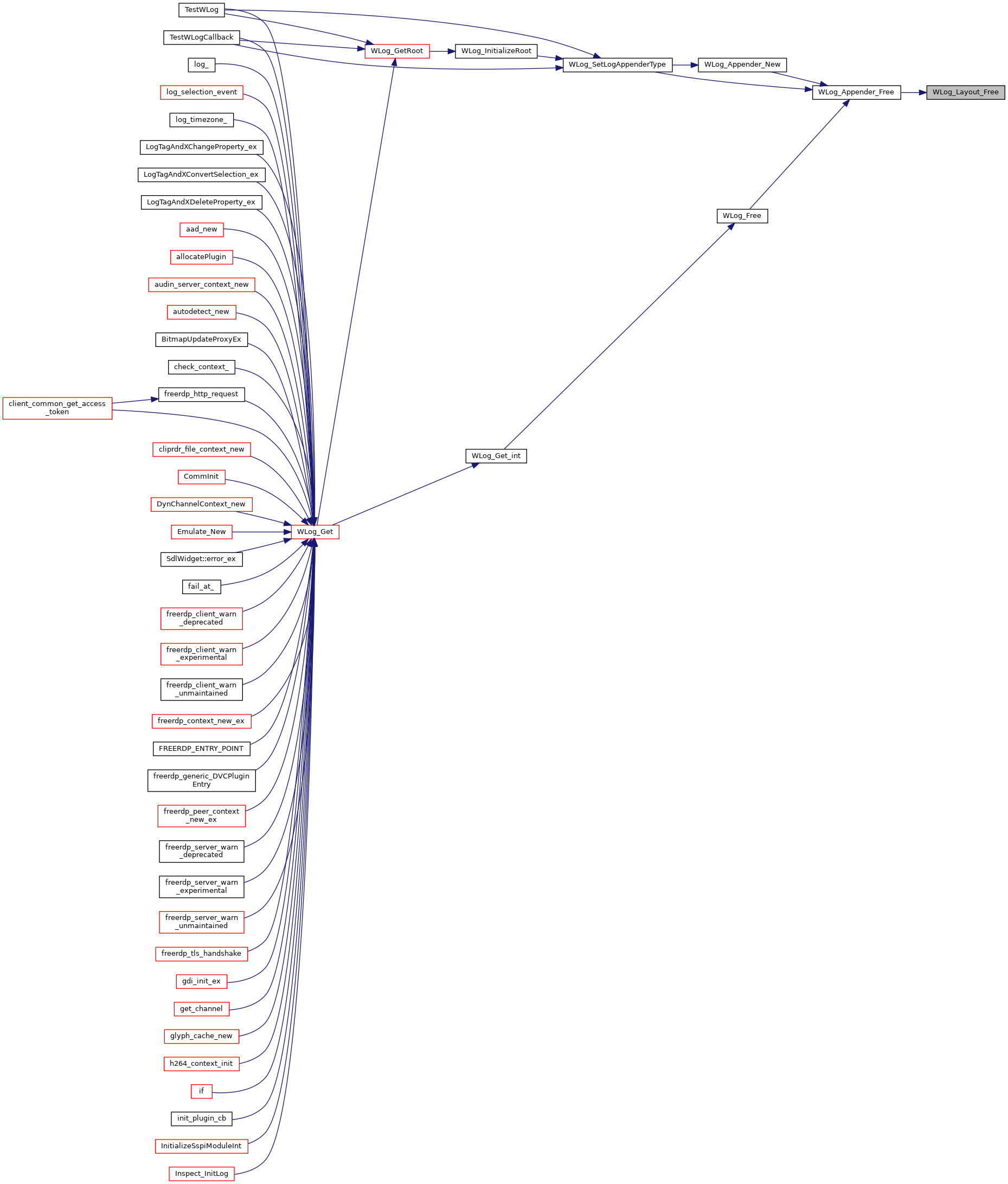BOOL WLog_Layout_GetMessagePrefix ( wLog * log, wLogLayout * layout, wLogMessage * message )

Here is the call graph for this function: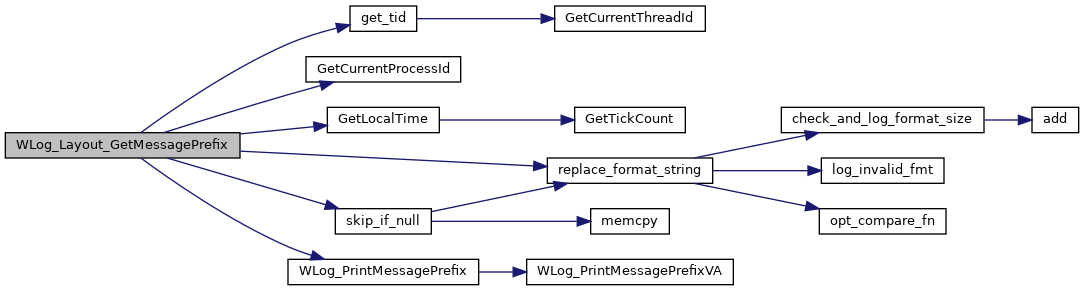Here is the caller graph for this function: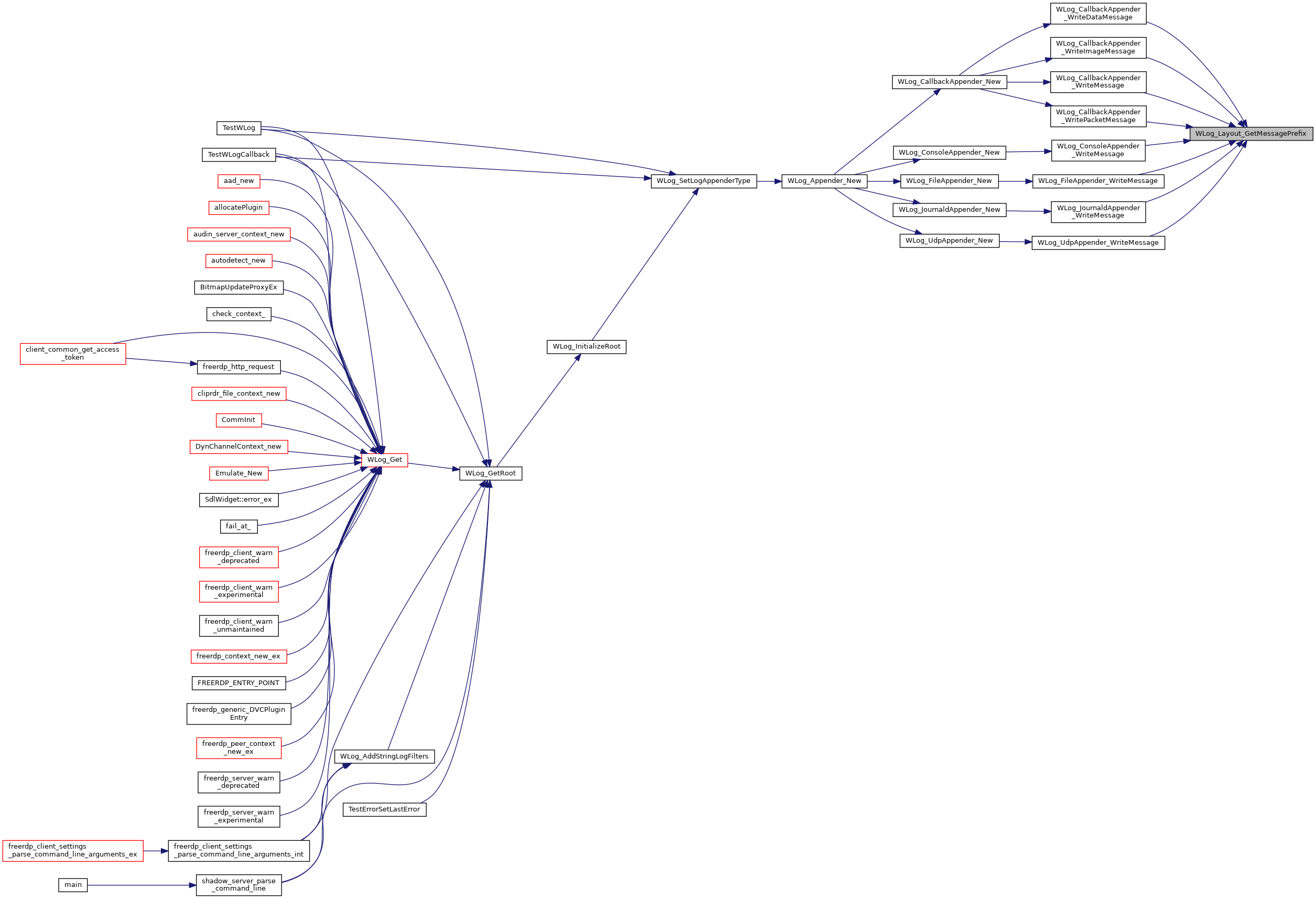wLogLayout* WLog_Layout_New ( wLog * log )

Here is the call graph for this function: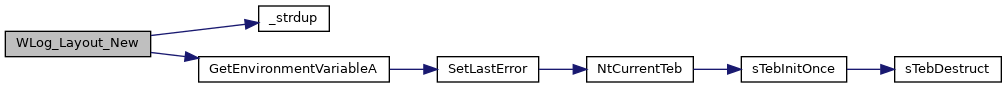Here is the caller graph for this function:BOOL WLog_Layout_SetPrefixFormat ( wLog * log, wLogLayout * layout, const char * format )

Here is the call graph for this function: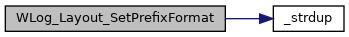Here is the caller graph for this function: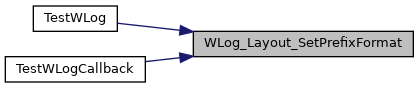static void WLog_PrintMessagePrefix ( wLog * log, wLogMessage * message, const char * format, ... )
static

Here is the call graph for this function: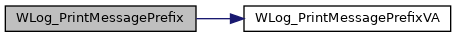Here is the caller graph for this function: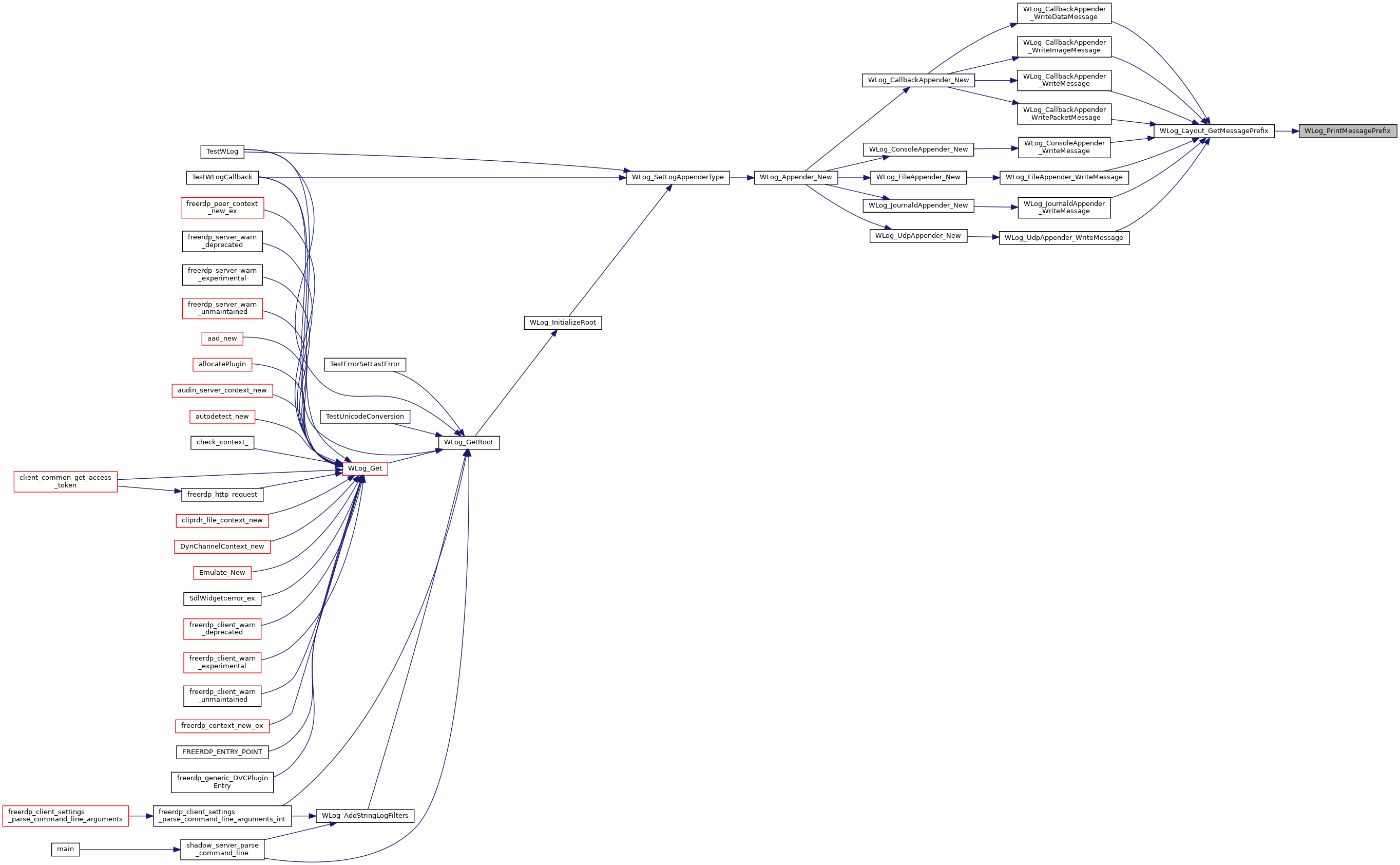static void WLog_PrintMessagePrefixVA ( wLog * log, wLogMessage * message, const char * format, va_list args )
static

Log Layout

Here is the call graph for this function: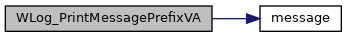Here is the caller graph for this function: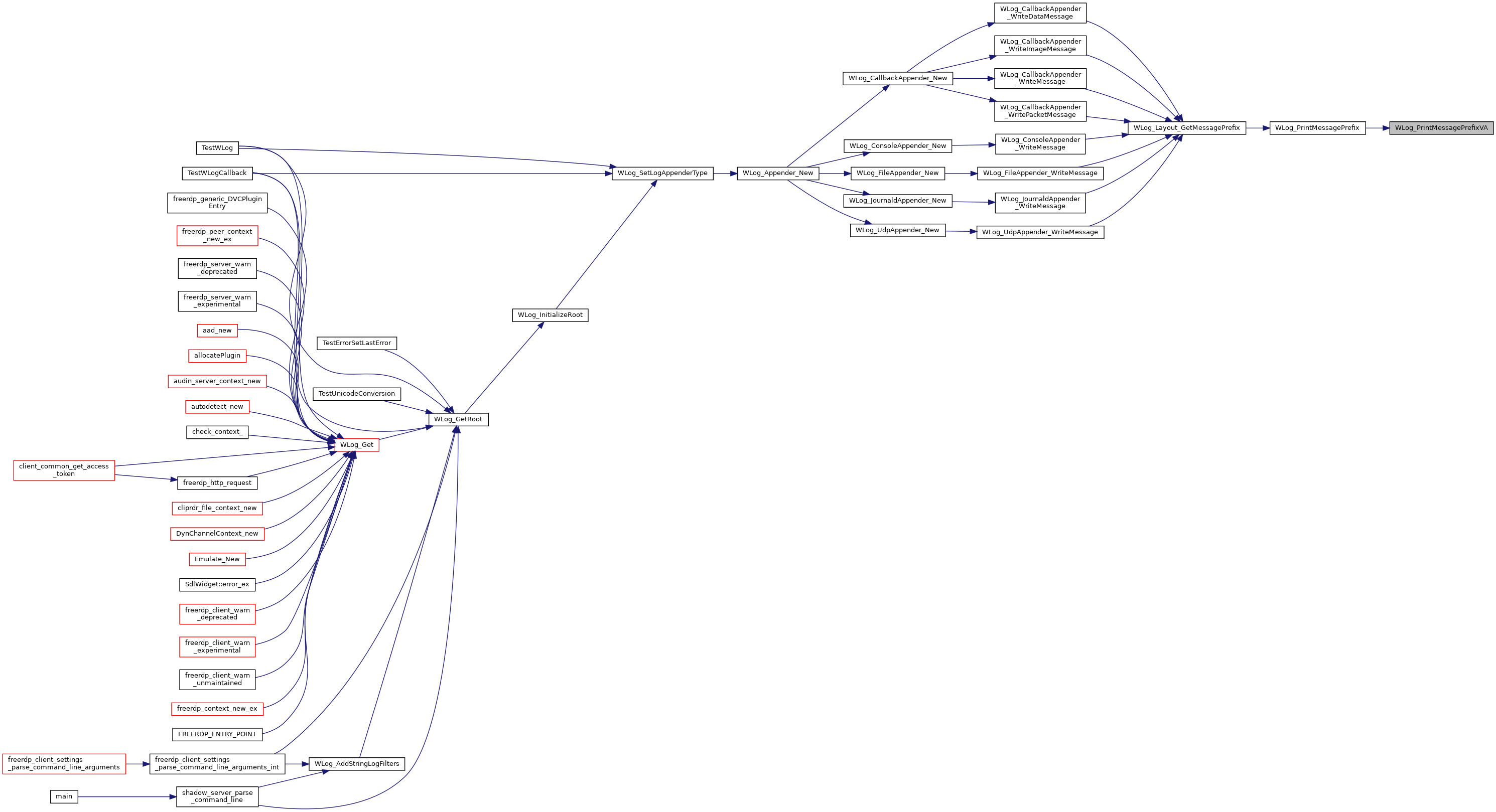Variable Documentation

 const char* WLOG_LEVELS

WinPR: Windows Portable Runtime WinPR Logger

```http://www.apache.org/licenses/LICENSE-2.0